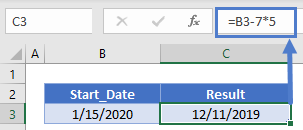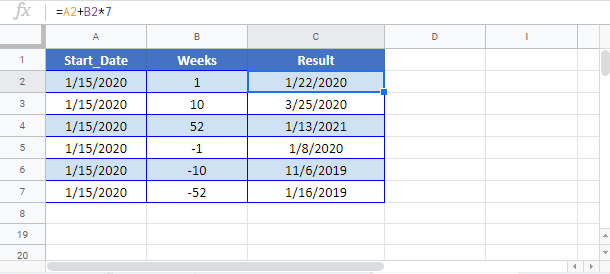Add or Subtract Weeks to a Date in Excel & Google Sheets

This tutorial will demonstrate how to add (or subtract) weeks in Excel & Google Sheets.Excel stores dates as serial numbers; each whole number representing an unique day.  That makes adding days to dates incredibly easy.  To add weeks to a date, we will add in 7-day increments.

This example will add 1 week (7 days) to a date: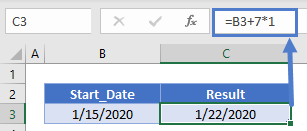Add 5 Weeks
This example will add 5 weeks (7*5=35 days) to a date: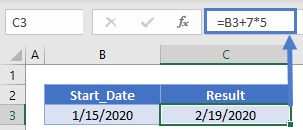Subtract Weeks From a Date
We can subtract weeks in the same way.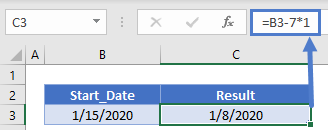Subtract 5 Weeks

This example will subtract 5 weeks (7*5=35 days) from a date: